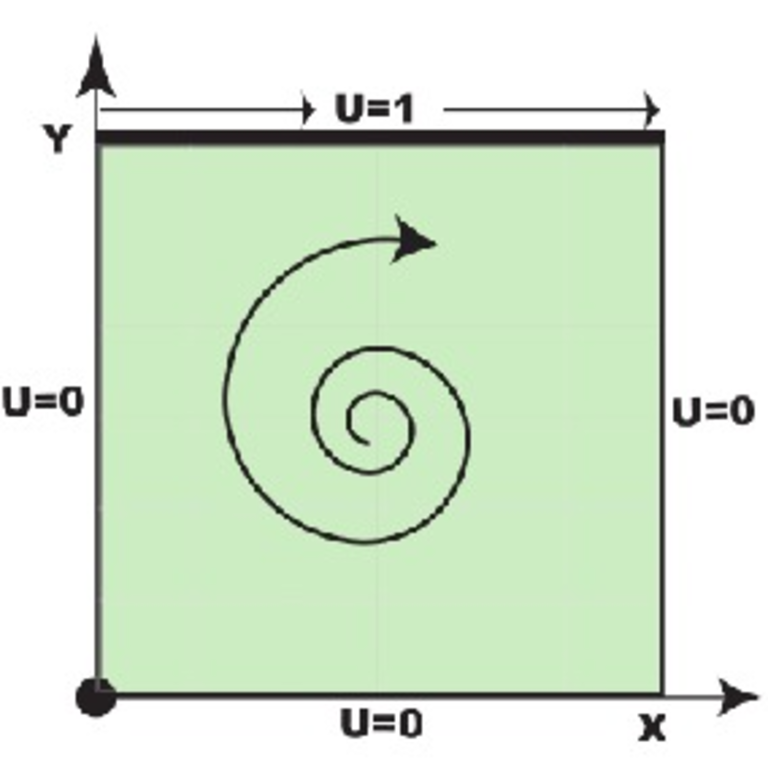## Test Case Setup

• We assume two-dimensional flow in cavity. Test case geometry is following:Figure: Lid driven cavity: Test case geometry.

• Test case has following parameters:
• Top wall velocity: U = 1 m/s
• Cavity edge length: d = 10 cm
• Laminar, Re = 10
• Incompressible
• Isothermal flow